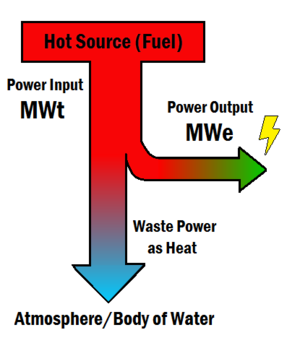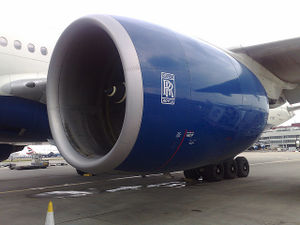Mechanical powerFigure 1. The power input in a heat engine is measured as MWt, and the output power obtained as electricity is measured as MWe. The ratio of power out over power in is the efficiency.Figure 2. A jet turbine can output a lot of work in a short amount of time, therefore having high mechanical power.

Mechanical power refers to the rate at which work can be done. It is a power output, as opposed to a power input (see Figure 1). The power input is referring to how fast the fuel's energy is converted to power to use for the car. In contrast, the power output, is how fast the engine can do work, when receiving the power from the fuel. The rate at which an engine uses fuel is the thermal power. Mechanical power is how fast mechanical energy can be delivered to a system. Recall that power is a transfer of energy in a specific amount of time.

Mechanical power is often measured in horsepower, although it's sometimes measured in watts. Some examples:

• The engine of a car
• The engine of an airplane (see figure 2)
• Using a crane to lift heavy things

If mechanical power is from a heat engine like a power plant, it is limited by the second law of thermodynamics and the maximum amount of mechanical power is given by the Carnot efficiency. Mechanical power of a wind turbine is also limited, although in a totally different aspect, by the Betz limit.

Simple machines are idealized as lossless. This means they do not lose any energy in their manipulation of forces. Therefore, mechanical power is conserved throughout the duration of its output, and this allows them to be analyzed easily in terms of mechanical advantage.